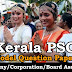## Recent Posts

1. 160m long train takes 8 seconds to cross a signal post. What is the speed of the train?
[a] 48km/hr
[b] 96km/hr
[c] 72 km/hr
[d] 84km/hr

2. If 10 miles equal 16 kilometers, how many kilometers make 7.5 miles?
[a] 10km
[b] 11km
[c] 12km
[d] 15km

3. In how many years a sum of money doubles in simple interest if rate of interest is 12%
[a] 8 years
[b] 12 years
[c] 10 years
[d] None of these

4. 27:257::87:_____?
[a] 457
[b] 675
[c] 818
[d] 857

5. 5% of (50% of 300) is
[a] 5
[b] 7.5
[c] 8.5
[d] 10

6. The population of a town increases 5% annually. If it is 15,435 now, What was it 2 years ago?
[a] 10000
[b] 11000
[c] 12000
[d] 14000

7. If wall is called window, window is called door, door is called floor, floor is called roof and roof is called ventilator, what will a person stand on?
[a] Window
[b] Wall
[c] Floor
[d] Roof

8. Mohan ranks 13th in a class of 31. What is his rank from the last?
[a] 15th
[b] 17th
[c] 19th
[d] 20th

9. 212, 179, 146, 113,_____
[a] 91
[b] 79
[c] 112
[d] 80

10. Showing the man receiving prize, seema said He is the brother of my uncles daughter. who is the man to seema?
[a] Son
[b] Brother -in -law
[c] Nephew
[d] Cousin

11. Jayesh travelled 4km straight towards south. He turned left and travelled 6 km straight then he turned right and travelled 4 km straight. How far is he from the starting point?
[a] 8km
[b] 10km
[c] 12km
[d] 18 km

12. If Midhun and Shyam together can complete a piece of work in 15 days and Shyam alone in 20 days. How much time shall Midhun take to complete the work?
[a] 60 days
[b] 40 days
[c] 30 days
[d] 50 days

13. A man makes a profit of 10% by selling a radio for Rs. 495. what is the cost price of the radio?
[a] 465
[b] 450
[c] 475
[d] 420

14. If 3A = 4B=6C, what is A:B:C?
[a] 3:4:6
[b] 6:4:3
[c] 4:3:6
[d] 4:3:2

15. The sum of the present ages of a father and his son is 60 years. Six years ago, fathers age was five times the age of the son. After 6 years, son`s age will be:
[a] 12 years
[b] 14 years
[c] 18 years
[d] 20 years

16. 16+4m =8, what is m?
[a] -4
[b] -8
[c] -2
[d] -12

17. Find the cost of painting the walls of a room 10 metres long, 5 metres broad and 6 metres high at the cost of Rs. 4 per square metre
[a] Rs. 720
[b] Rs. 880
[c] Rs. 360
[d] Rs. 1200

18. ELFA, GLHA, ILJA, _____, MLNA
[a] OLPA
[b] KLMA
[c] LLMA
[d] KLLA

19. A clock is started at noon. By 10 minutes past 5, the hour hand has turned through:
[a] 145º
[b] 150º
[c] 155º
[d] 160º

20. What was the day of the week on 28th May, 2006?
[a] Thursday
[b] Friday
[c] Saturday
[d] Sunday

Model Question Paper Company Corporation Board Assistant - 34Reviewed by Santhosh Nair on 1:38 PM Rating: 5